• 结构体排序
千次阅读
2022-03-27 15:57:39

## C++结构体排序（入门）

引入头文件后，定义排序规则！

#include<algorithm>
#include<string>
using namespace std;

typedef struct {
int score;
string name;
} stu;

bool cmp(stu a,stu b){
return a.score<b.score;
}// <升序  >降序

int main() {
stu s;
s.score=4;
s.score=3;
s.score=2;
s.score=1;
s.name="四";
s.name="三";
s.name="二";
s.name="一";
for(int i=0; i<4; i++) {
cout<<s[i].score<<" "<<s[i].name<<endl;
}
sort(s,s+4,cmp);
for(int i=0; i<4; i++) {	//加N就是对N个数排序！
cout<<s[i].score<<" "<<s[i].name<<endl;
}
return 0;
}c++
更多相关内容
• 用go语言实现的结构体排序，使用go的反射包（reflect），实现结构体按指定字段排序，字段类型目前支持int，int64，string，方便扩展任意基本类型，使用直接导入包，然后调用sortStruct(structArr, 'sorft_name', ...
• 针对结构体排序问题，分三步走：①声明结构体 ② 重新定义排序规则 ③主函数里面的读数据，利用sort()排序，及根据题意输出 以例题进行讲解：[NOIP2007 普及组] 奖学金 - 洛谷 这道题比较经典，题目较长，但不必...

针对结构体排序问题，分三步走：①声明结构体 ② 重新定义排序规则 ③主函数里面的读数据，利用sort()排序，及根据题意输出

以例题进行讲解：[NOIP2007 普及组] 奖学金 - 洛谷

这道题比较经典，题目较长，但不必害怕！！！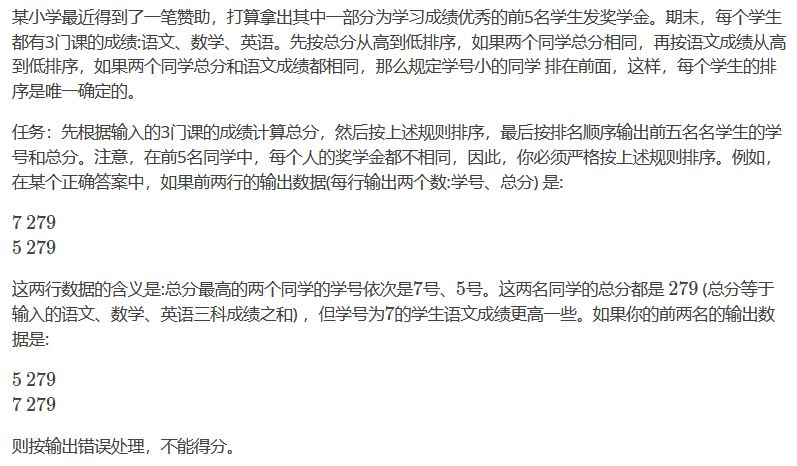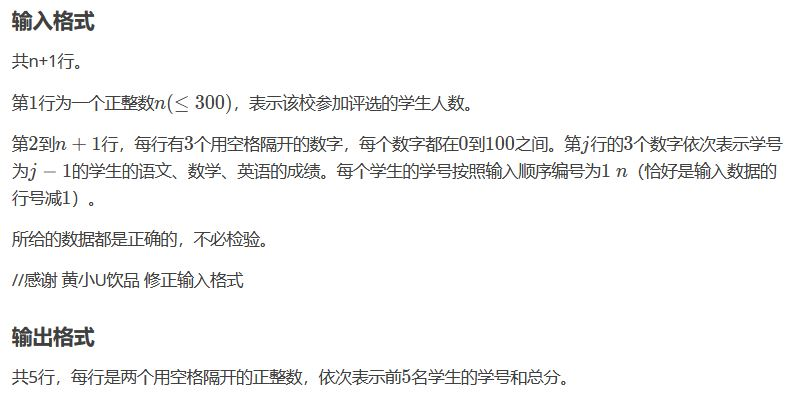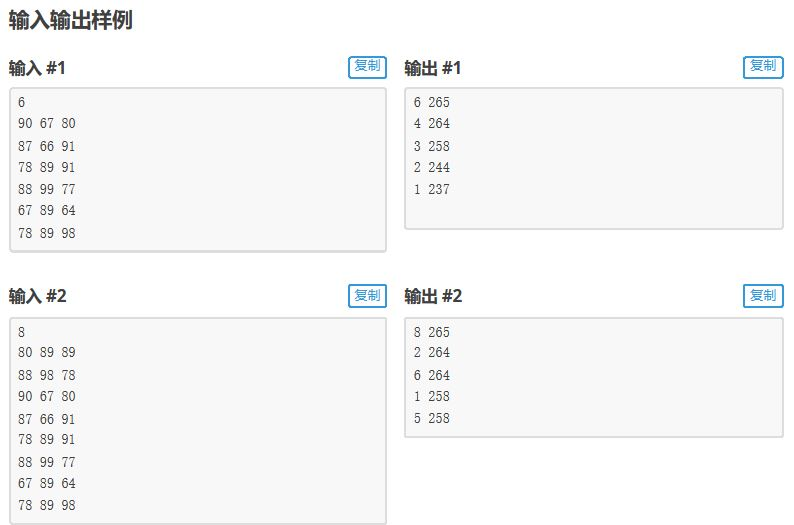输入：先是输入学生总人数，后面每一行分别代表语文，数学，英语的成绩。

输出 : 输出前5名学生的学号和总分。

①声明结构体，结构体里面定义的变量分别为：语文成绩，数学成绩，英语成绩，题目中还涉及总分，以及学号，故结构体声明如下：

#include <bits/stdc++.h>

using namespace std;

struct stu{
int id; //学号
int chinese; //语文成绩
int math;  //数学成绩
int english;  //英语成绩
int score;  //总分
};

②重新定义排序规则：依据题意我们可以得知排序规则：

（1）总分从高到低

（2）总分相同时，语文成绩从高到低排

（3）总分和语文成绩相同时，学号小的排在前面。

bool cmp(stu a, stu b){
if(a.score != b.score){ //总分不同时，从高到低排
return a.score > b.score;
}else{ //总分相同时
if(a.chinese != b.chinese){ //语文成绩不同，从高到低排
return a.chinese > b.chinese;
}else{ //总分和语文成绩相同时，学号小的往前排
return a.id < b.id;
}
}
}

③主函数（读，sort排序，输出）：

int main(){
int n;
cin >> n;
stu s[n]; //定义结构体数组
for(int i = 0; i < n; i++){
int a, b, c;
cin >> a >> b >> c; //a b c代表语文，数学，英语成绩
int d = a + b + c; //d 代表总分
s[i] = {i+1,a,b,c,d}; //结构体变量里面的每一个分量的值
}
sort(s,s+n,cmp); //利用sort排序
//按照题意进行输出
for(int i = 0; i < 5; i++){
cout << s[i].id << " " << s[i].score << endl;
}
return 0;

}

总代码：

#include <bits/stdc++.h>

using namespace std;

struct stu{
int id;
int chinese;
int math;
int english;
int score;
};
bool cmp(stu a, stu b){
if(a.score != b.score){
return a.score > b.score;
}else{
if(a.chinese != b.chinese){
return a.chinese > b.chinese;
}else{
return a.id < b.id;
}
}
}
int main(){
int n;
cin >> n;
stu s[n];
for(int i = 0; i < n; i++){
int a, b, c;
cin >> a >> b >> c;
int d = a + b + c;
s[i] = {i+1,a,b,c,d};
}
sort(s,s+n,cmp);
for(int i = 0; i < 5; i++){
cout << s[i].id << " " << s[i].score << endl;
}
return 0;

}

结构体类型的题目其实是简单的，多做几道题目就好，刚开始的时候我也不明白。

在做题过程中，你需知道如何声明结构体，排序规则，以及数据的读取，排序，输出即可。

我上面写的代码是可以作为模板的，按照这个套路写就好。

类题如下：

生日 - 洛谷

[NOIP2009 普及组] 分数线划定 - 洛谷

宇宙总统 - 洛谷 （这道题与其他题有点不同，排序对象是字符串）

[NOIP2005 提高组] 谁拿了最多奖学金 - 洛谷（这道题绕的弯较多，弄清楚排序规则就好）

我把每道题目的代码以单独的文章贴出，大家可以进行一个对比。

展开全文算法
• ## python结构体排序

千次阅读 2022-01-25 19:31:11
在c/c++中，遇到结构体排序类似的问题时可以直接用struct定义一个结构体，然后重写cmp函数实现结构体排序，而在python中，没有结构体，那么如何实现结构体排序？ 在python中，列表有两种排序方式，分别是sort和...

### 文章目录

在c/c++中，遇到结构体排序类似的问题时可以直接用struct定义一个结构体，然后重写cmp函数实现结构体排序，而在python中，没有结构体，那么如何实现结构体排序？

在python中，列表有两种排序方式，分别是sortsorted

• sort是列表的内置方法，可以直接调用，会修改原列表并排好序默认升序，可以通过reverse=True实现降序。cmp函数是比较方法，在python3中已经被废除，key可以指定比较的参数，一维列表一般不需要写key。
list.sort(cmp=None, key=None, reverse=False)

• sorted会新建一个排序好的列表并返回，默认升序，可以通过reverse=True实现降序。同样cmp在python3中已经被废除，key可以指定比较的参数。
sorted(iterable, cmp=None, key=None, reverse=False)


下面介绍如何实现类似于c/c++中的结构体排序。

结构体就是自定义的数据类型，在一个结构体中可以存储任意类型数据。
而列表中可以存储任意类型的数据，可以用一个二维列表充当结构体。

### 一个参数比较

第一种情况，例如将姓名和成绩作为一个列表保存至一个二维列表中，输入每个学生的姓名和成绩，按照学生成绩降序排除，这种情况可以使用sort函数中的key来指定比较的参数。

①写一个getKey方法

def getKey(x):
return int(x)


②调用sort函数时，将getKey函数传入

def getKey(x):		# 按照下标为1的元素进行排序
return int(x)

N=int(input())
list=[]
for i in range(N):
item=input().split()	#输入姓名和成绩
list.append(item)

list.sort(key=getKey,reverse=True)
print(list)


也可以用lambda表达式来写

# 表示按x升序排序
list.sort(key=lambda x:x)


下面这个题目就可以使用这种方法来做
第K名

刚举行的万米长跑活动中，有 NNN 个人跑完了全程，所用的时间都不相同。颁奖时为了增加趣味性，随机抽了一个数K，要奖励第 K 名一双跑鞋。
现在组委会给你 N 个人的姓名、成绩（用时，单位是秒），请你编程快速输出第 K 名的姓名。

输入格式：
第一行：2 个整数 N 和 K。
下面 N 行：每行第 1个是字符串表示姓名；第 2 个是个整数，表示这个人跑完的使用时间。
输出格式：
一行，第 K 名的姓名。

代码：

def getKey(x):
return int(x)

N,K=map(int,input().strip().split())
list=[]
for i in range(N):
item=input().split()
list.append(item)

list.sort(key=getKey)
# 下边是lambda表达式写法
# list.sort(key=lambda x:int(x))
print(list[K-1])



### 多个参数比较

第二种情况比如，列表中有三个数据，首先比较第一个数据，如果第一个数据相等，那么比较第二个，以此类推，这时，通过指定key就无法实现排序了，就必须写一个cmp函数来实现，但是python3又将cmp函数废除了，这时可以通过functools库下的cmp_to_key来实现，然后调用sort时将cmp函数传入，具体看下面一个题目

日期排序

有一些日期，日期格式为 “MM/DD/YYYY”。
编程将其按日期大小排列。

输入格式：
输入第一行一个整数 n(1≤n≤1000)，表示日期的个数。
接下来 n 行按照题目描述的格式输入 n 个日期。
输出格式：
输出从早到晚排序后的日期，一个日期占一行，日期输出的格式和输入一样。

代码：

from functools import cmp_to_key
def mycmp(a,b):
if a>b:
return 1
elif a==b:
if a>b:
return 1
elif a==b:
if a>b:
return 1
else:
return -1
else:
return -1
else:
return -1

list=[]
n=int(input())
for i in range(n):
item=input()
a=[]
a.append(int(item[0:2]))
a.append(int(item[3:5]))
a.append(int(item[6:]))
list.append(a)

list.sort(key=cmp_to_key(mycmp))
# 也可以这么写，先按x升序，然后按x升序，然后按x升序
#list.sort(key=lambda x:(x,x,x))

for item in list:
if item<10:
print(0,end="")
print("{}/".format(item),end="")
if item<10:
print(0,end="")
print("{}/".format(item),end="")
print(item)



对于cmp函数的写法，这里举例一个简单的，如果传入的参数a和b，首先比较a和b，如果a>b，返回1，则表示以第一个元素按升序排序，如果返回-1，则是降序排序，当a==b时，开始比较第二个元素，如果a>b，返回-1，则表示第一个元素相等，第二个元素按降序排序。

这个cmp函数表示，按第一个元素的升序和第二个元素的降序进行排序

def mycmp(a,b):
if a>b:
return 1
elif a==b:
if a>b:
return -1
else:
return 1
else:
return -1


调用sort时，将cmp传入即可，注意需要导相应的库

from functools import cmp_to_key

list.sort(key=cmp_to_key(mycmp))


例如：

from functools import cmp_to_key
def mycmp(a,b):
if a>b:
return 1
elif a==b:
if a>b:
return -1
else:
return 1
else:
return -1

list=[[5,0],[5,8],[3,5],[5,2],[0,3],[0,5]]
list.sort(key=cmp_to_key(mycmp))
print(list)

# 输出：[[0, 5], [0, 3], [3, 5], [5, 8], [5, 2], [5, 0]]


上边写法过于复杂，可以直接使用lambda表达式来写

#先按x升序，然后按x升序，然后按x升序
list.sort(key=lambda x:(x,x,x))

展开全文python 算法
• ## 结构体排序+sort()

千次阅读 2021-11-13 10:59:30
1.结构体定义 结构体(struct)是由一系列具有相同类型或不同类型的数据构成的数据集合，也叫结构。 通俗的来说其实就是，开辟一个新的变量来存储这个变量的不同属性，比如，学生成绩，它有语数外三科成绩，因为这...

目录

1.结构体定义

2.sort排序

1.sort()函数的头文件和用法举例

2.sort（）函数的参数说明

两个参数

三个参数

3.结构体排序

# 1.结构体定义

结构体(struct)是由一系列具有相同类型或不同类型的数据构成的数据集合，也叫结构。

通俗的来说其实就是，开辟一个新的变量来存储这个变量的不同属性，比如，学生成绩，它有语数外三科成绩，因为这三科成绩属于同一个人。

struct student{
int Ch;//语文
int math;//数学
int En;//英语
}p;

后面是一个可选的标志（p），它是用来引用该结构体的快速标记.

这里的我开了一个大小为10000的数组，表示开辟了一个可以存下10000个学生成绩那么大的结构体数组。

# 2.sort排序

结构体排序，我这里介绍调用sort函数的这一种排序；

## 1.sort()函数的头文件和用法举例

首先要调用sort函数，一定要加上这个头文件  #include<algorithm>

其实就和你要调用pow()函数一定要加上#include<cmath>头文件一个道理

用法举例如下：

#include<iostream>
#include<algorithm>
using namespace std;
int main()
{
int a={1,4,6,3,2};
sort(a,a+5);
for(int i=0;i<5;i++)
cout<<a[i]<<" ";
cout<<endl;
return 0;
}

输出结果如下：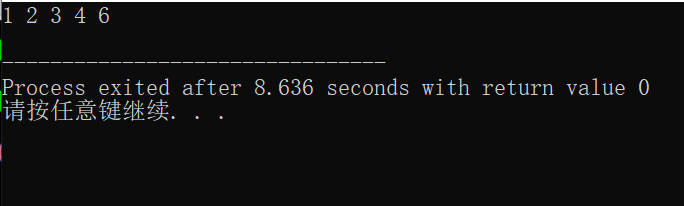## 2.sort（）函数的参数说明

sort函数可以是两个参数，也可以是三个参数，这里我们先介绍两个参数的那种情况，在介绍三个参数的情况；

### 两个参数

也就是sort函数没有第三个参数，这种情况就是，默认实现的是从小到大，也就是刚刚举例的那种情况

#include<iostream>
#include<algorithm>
using namespace std;
int main()
{
int a={9,6,3,8,5,2,7,4,1,0};
for(int i=0;i<10;i++)
cout<<a[i]<<" ";//打印排序之前的数组序列
cout<<endl;
sort(a,a+10);//这里调用sort()函数
for(int i=0;i<10;i++)
cout<<a[i]<<" ";//打印排序之后的数组序列
return 0;
}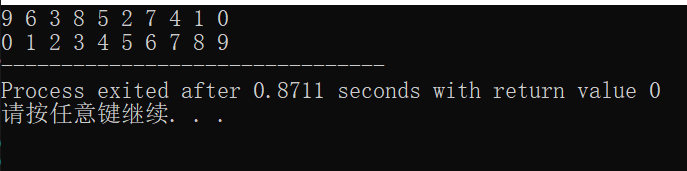### 三个参数

Sort函数有三个参数：

（1）第一个是要排序的数组的起始地址。

（2）第二个是结束的地址（最后一位要排序的地址）

（3）第三个参数是排序的方法，可以是从大到小也可是从小到大，还可以不写第三个参数，此时默认的排序方法是从小到大排序。

【注意】这里的第三个参数就是自己定义的比较方式的函数，比如下面的例子我定义的函数名字是cmp，（是bool类型），我这个函数返回的是 a>b,所以这里调用了sort之后数组里的元素按照从小到大的顺序排列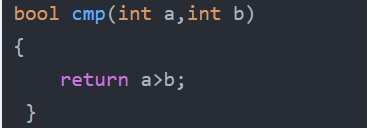Sort函数使用模板:

Sort(start,end,排序方法)

代码如下：自己可以尝试一下写一个类似的代码，运行一下：哈哈哈👀ヾ(•ω•)oヾ(•ω•)o

#include<iostream>
#include<algorithm>
using namespace std;
bool cmp(int a,int b)
{
return a>b;
}
int main() {
int a= {9,6,3,8,5,2,7,4,1,0};
cout<<"最原始的数组：";
for(int i=0; i<10; i++)
cout<<a[i]<<" ";
cout<<endl;
sort(a,a+10,cmp);
cout<<"调用三个参数sort()函数排序后的数组：";
for(int i=0; i<10; i++)
cout<<a[i]<<" ";
cout<<endl;
sort(a,a+10);
cout<<"调用两个参数sort()函数排序后的数组：";
for(int i=0; i<10; i++)
cout<<a[i]<<" ";
return 0;
}

输出结果：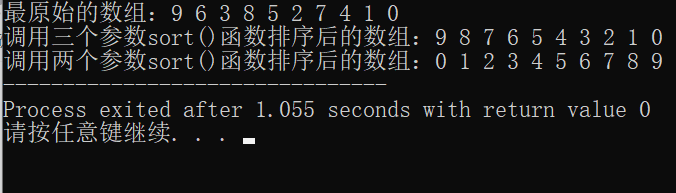# 3.结构体排序

之前说的结构体，就是包含了多个元素的变量，，然后，，，这里讲到的sort函数就是对数组里面的元素进行排序，那么如果这是一个结构体数组，我们也可以用sort函数，对这个结构体数组进行类似的操作了

来看个题目吧：
某小学打算为学习成绩优秀的前5名学生发奖学金。期末，每个学生都有3门课的成绩:语文、数学、英语。总分从高到低排序，如果两个同学总分相同，语文成绩从高到低排序，如果两个同学总分和语文成绩都相同，那么规定学号小的同学 排在前面，这样，每个学生的排序是唯一确定的。
任务：先根据输入的3门课的成绩计算总分，然后按上述规则排序，最后按排名顺序输出前五名名学生的学号和总分。注意，在前5名同学中，每个人的奖学金都不相同，因此，你必须严格按上述规则排序。例如，在某个正确答案中，如果前两行的输出数据(每行输出两个数:学号、总分) 是:
7 279
5 279
这两行数据的含义是:总分最高的两个同学的学号依次是7号、5号。这两名同学的总分都是 279 (总分等于输入的语文、数学、英语三科成绩之和) ，但学号为7的学生语文成绩更高一些。如果你的前两名的输出数据是:
5 279
7 279
则按输出错误处理，不能得分。

传送门：查看完整题目

看完题目得知，这里有很多个学生，每个学生有自己的 三门成绩，语数英，还有学号，那我们得定义一个结构体数组，把每个学生的这些信息储存起来，再按照规定进行排序，输出前五名就是我们要完成的任务

话不多说，上代码：

#include<iostream>
#include<algorithm>
using namespace std;
struct student{
int c;//语文分数
int s;//数学分数
int y;//英语分数
int num;//学号
int sum;//每个人的总分
}p;
bool cmp(const student&a,const student&b)
{
if(a.sum!=b.sum)//如果总分不相同，就返回总分高的那一个
return a.sum>b.sum;
else if(a.sum==b.sum&&a.c!=b.c)//如果总分相同，就返回总分语文高的那一个
return a.c>b.c;
else if(a.sum==b.sum&&a.c==b.c&&a.num!=b.num)
//如果总分相同，语文分相同，就返回总分学号小的那一个
return a.num<b.num;
}
int main()
{
int n;
cin>>n;
for(int i=0;i<n;i++)
{
cin>>p[i].c>>p[i].s>>p[i].y;//输入信息
p[i].num=i+1;//序号即学号
p[i].sum=p[i].c+p[i].s+p[i].y;//算出每个人的总分
}
sort(p,p+n,cmp);//排序
for(int i=0;i<5;i++)//输出前五名的学号和总分
cout<<p[i].num<<" "<<p[i].sum<<endl;
return 0;
}

这样就可以完成任务了。

拿去吧，去给皇上看，你告诉他，这都是本宫自己敲的，没有假手于人，本宫手都要断了。

ಥ_ಥ

展开全文算法
• #include "stdafx.h"#include#include#includeusing namespace std;struct student{string name;//姓名int math;...//控制排序规则bool compare(student a, student b){if (c == 0)//按照姓名递增排序...
• 结构体排序的两种实现方法 1.使用sort函数，重写排序规则。 #include<iostream> #include<algorithm> #include<queue> using namespace std; struct node{ int x; int y; }a; bool ...c++ 算法
• 题目大意：从一定数量的参加笔试的人中挑出符合面试条件的人，面试的条件是按照成绩从大到小排序，在需要录取的人数的150%处截取，截取的笔试分数就是可以参加面试的条件。（如果截取分数处有好几个人都是这个分数，...c++ 算法
• 一、模仿C语言的结构体读入数据 class student: # 固定格式 def __init__(self): # 固定格式 self.name = "" self.num = "" self.age = "" list1 = [] # 创建空列表来准备存储 for i in range(0, 3): list1....python
• #include using namespace std; typedef struct { string book; int num; }Book; //qsort的比较函数 int cmp(const void * a, const void * b) { return (*(Book*)a).num > (*(Book*)b).num ?...
• #include <string> #include <vector> #include <iostream> #include <algorithm> using std::string; using std::vector; using std::cout; using std::endl;...bool GreaterS.
• ## C++ 结构体排序

千次阅读 2019-11-27 20:53:51
C++ 结构体排序 引入头文件 #include<algorithm> 结构体 bool compare(const node &x, const node &y) { return x.v > y.v; } 当用 大于号就是从大到小排序 用小于号就是从小到大排序 sort 函数...c++
• 结构体排序：写法一QList<test> s; test aa; test bb; test cc; aa.num = "14"; bb...字符串 列表 leetcode latex
• ## go语言结构体排序

千次阅读 2020-08-27 10:36:59
2. 结构体排序需要实现三个函数（获取长度函数，交换函数，比较函数）即可 实验如下图 源代码如下 package main import ( "fmt" "sort" ) // 学生信息 type Student struct { Age int Score int } // ...
• ## 结构体排序（sort）

千次阅读 多人点赞 2021-11-04 11:12:55
个人比较喜欢用结构体来存两个有关联的变量，而不喜欢用二维数组，因为很容易出错，所以，想写一篇关于结构体排序的博客哈哈哈哈哈✪ ω ✪ 头文件 我最经常用的是sort排序,,,这里头文件用的是 #include<...算法
• 文章目录基本类型 int 、 float64 和 string 的排序升序排序降序排序深入理解排序结构体类型的排序结构体排序方法 1结构体排序方法 2结构体排序方法 3结构体排序方法 4 晚上准备动手写点 go 的程序的时候，想起 go ...golang
• 结构体内部重载小于号， #include <iostream> #include <algorithm> using namespace std; struct Student { string name; int MathGrade; int ChineseGrade; //重载小于号，语文成绩大的学生排...
• Golang对结构体字段排序的案例 本案例通过 sort 方法,对产品的 price 字段进行排序,仅供参考... package main import ( "fmt" "sort" ) type Product struct { Name string Price float64 Date string } ...golang
• \n",people) sort.Sort(PersonSlice(people)) fmt.Println("按照学费从低到高排序的结果\n", people) // 按照年龄从低到高排序 sort.Slice(people, func(i, j int) bool { return people[i].Age [j].Age }) ...go
• 自己理解的，结构体是一种可以自己定义一个新的“型”的神奇的东西，而它的特征就是里面可以放上各种奇奇怪怪的东西（就是各种各样的型，比如int，float，char啥的），让它们联系起来。。。 emmm，其实这玩意的定义...
• ## C语言结构体和结构体排序

千次阅读 多人点赞 2019-01-26 22:06:48
结构体 结构体是一种构造数据类型 （构造数据类型包括：数组类型、结构体类型、共用体类型） 定义：把不同的数据组合成一个整体。 结构体的一般形式 struct [结构体名称]//struct是关键字 { 类型标识符 成员名；//...
• {CreatedAt: time.Now().Add(500 * time.Hour)}, } for _, v := range hh { fmt.Printf("%#v\n", v.CreatedAt.Format("2006-01-02 15:04:05")) } fmt.Println("排序后") sort.Sort(hh) for _, v := range...
• C++中自定义结构体选择一个键值 调用sort qsort排序
• //定义一个结构体将名字和分数捆绑在一起 struct student { char name ; int score; }; int main () { struct student a,t; int i,j,n; scanf("%d",&n); for (i=1;i<=n;i++)//循环读入n个人名...c语言 开发语言 后端
• ## C++中的结构体排序

千次阅读 2019-10-08 13:48:38
最近在上算法课程，老师要求用C++来做，刚好软件开发选的也是C++（虽然自己选的方向是Java）...struct是结构体的关键字，Example相当于结构体的一个主体，定义变量可以如下： Example exam; 2 typedef struct{ in...C++
• 在写算法时，若要对自定义的结构体或类进行排序，常常以重载运算符的方式，加上标准模板库里的sort()函数进行排序。但是有时我们需要排序的对象是结构体的指针，这时就要使用其他方法。本文记录我的使用的两种算法...c++ 重载运算符 sort 模板...